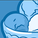Announcements
Applications are open for the 2024 Qlik Luminary Program. Apply by December 15 here.
cancel
Showing results for
Did you mean:Contributor

## If Statement and Set analysis in Pivot Table: excluding a Dimension leads to a wrong calculation.

Dear Experts!

I've been struggling for two days without results, therefore I ask support to the community.

I have a Pivot Table where the Measure is calculated based on a SET ANALYSIS

On Top of it, the measure should be calculated multipling by -1  based on the VALUE of a DIMENSION.

The expression of the Measure looks similar to this (I'll over-simplify the whole expression, this is not the actual code)

=IF(DIMENSION  = 'VALUE'), -1, 1) * (SET ANALYSIS)

Everything works fine if I add the DIMENSION as first dimension of my Pivot Table.

The problem is I need to hide (better totally exclude) the DIMENSION in the Pivot. In this case the "if" statement above  fails, and the results have all positive sign.

Below the Details:

=IF(AT = 'RE'), -1, 1) * Sum({<SA = {'PL'}>*<LL = {'II7'}>*<T= {'[NONE]'}>*<AT -= {'AS'}>*<AT -= {'LI'}>} AMOUNT)

Any idea how to fix this?

RP

3 RepliesSpecialist

You need to use the Aggr() function.  Something like this:

sum(aggr(if(AT='RE',-1,1)*sum({<SA = {'PL'}>*<LL = {'II7'}>*<T= {'[NONE]'}>*<AT -= {'AS'}>*<AT -= {'LI'}>} Amount), AT))Contributor
Author

Thanks for your comment! trying with aggr(), the expressions has no errors but unfortunately I'm not getting the desired results.Partner - Specialist

Hi @RP8 , I think what's happening is, if you include the dimension in the table, the If() condition is evaluated for every row and every row has a different AT value, so it works. But if you exclude the dimension from the table, AT is not a single value (unless you select only one), so you should use an aggregation function to get only one value for your comparison, for example:

IF(MaxString(AT) = 'RE'), -1, 1) * Sum({<SA = {'PL'}>*<LL = {'II7'}>*<T= {'[NONE]'}>*<AT -= {'AS'}>*<AT -= {'LI'}>} AMOUNT)

JGTags
Community Browser